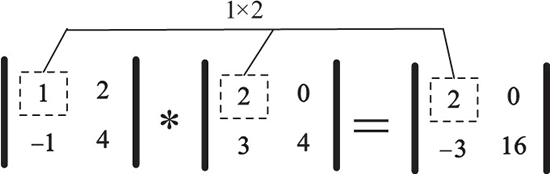## NumPy的算术运算

• 内容
• 评论
• 相关

• 一种是对应元素相乘，又称为逐元乘法（Element-Wise Product），可以使用 np.multiply() 函数或者`*`运算符；
• 另一种是点积或内积元素，运算符为 np.dot()。

## 对应元素相乘

numpy.multiply(x1, x2, /, out=None, *, where=True,casting='same_kind', order='K', dtype=None, subok=True[, signature, extobj])

```>>> A = np.array([[1, 2], [-1, 4]])
>>> B = np.array([[2, 0], [3, 4]])
>>> A*B
array([[ 2,  0],
[-3, 16]])
>>> np.multiply(A, B)
array([[ 2,  0],
[-3, 16]])```0条评论